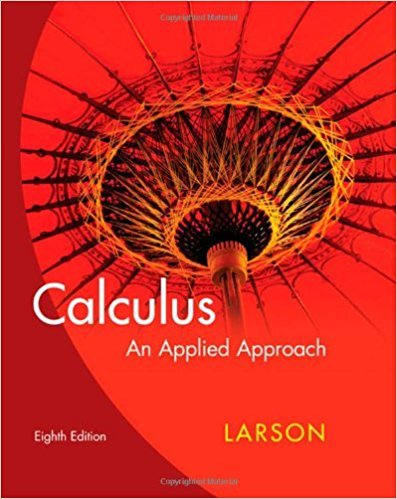×
×

# Solutions for Chapter 2.8: Related Rates## Full solutions for Calculus: An Applied Approach | 8th Edition

ISBN: 9780618958252Solutions for Chapter 2.8: Related Rates

Solutions for Chapter 2.8
4 5 0 411 Reviews
22
1
##### ISBN: 9780618958252

Since 26 problems in chapter 2.8: Related Rates have been answered, more than 22801 students have viewed full step-by-step solutions from this chapter. Chapter 2.8: Related Rates includes 26 full step-by-step solutions. This expansive textbook survival guide covers the following chapters and their solutions. This textbook survival guide was created for the textbook: Calculus: An Applied Approach , edition: 8. Calculus: An Applied Approach was written by and is associated to the ISBN: 9780618958252.

Key Calculus Terms and definitions covered in this textbook

P(A or B) = P(A) + P(B) - P(A and B). If A and B are mutually exclusive events, then P(A or B) = P(A) + P(B)

• Boxplot (or box-and-whisker plot)

A graph that displays a five-number summary

• Cubic

A degree 3 polynomial function

• Equation

A statement of equality between two expressions.

• equation of a hyperbola

(x - h)2 a2 - (y - k)2 b2 = 1 or (y - k)2 a2 - (x - h)2 b2 = 1

• Independent variable

Variable representing the domain value of a function (usually x).

• Inequality symbol or

<,>,<,>.

• Linear correlation

A scatter plot with points clustered along a line. Correlation is positive if the slope is positive and negative if the slope is negative

• Mapping

A function viewed as a mapping of the elements of the domain onto the elements of the range

• Median (of a data set)

The middle number (or the mean of the two middle numbers) if the data are listed in order.

• Multiplicative inverse of a complex number

The reciprocal of a + bi, or 1 a + bi = a a2 + b2- ba2 + b2 i

• nth root of a complex number z

A complex number v such that vn = z

• One-to-one function

A function in which each element of the range corresponds to exactly one element in the domain

• One-to-one rule of logarithms

x = y if and only if logb x = logb y.

• Polar coordinate system

A coordinate system whose ordered pair is based on the directed distance from a central point (the pole) and the angle measured from a ray from the pole (the polar axis)

• Position vector of the point (a, b)

The vector <a,b>.

An equation that can be written in the form ax 2 + bx + c = 01a ? 02

• Remainder theorem

If a polynomial f(x) is divided by x - c , the remainder is ƒ(c)

• Root of a number

See Principal nth root.

• Symmetric matrix

A matrix A = [aij] with the property aij = aji for all i and j

×